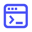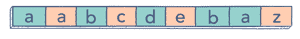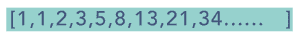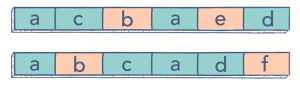# 6 Dynamic Programming problems for your next coding interview

Jan 15, 2019 - 14 min readEducativeMany programmers dread dynamic programming (DP) questions in their coding interviews. It’s easy to understand why. They’re hard!

For one, dynamic programming algorithms aren’t an easy concept to wrap your head around. Any expert developer will tell you that DP mastery involves lots of practice. It also requires an ability to break a problem down into multiple components, and combine them to get the solution.

Another part of the frustration also involves deciding whether or not to use DP to solve these problems. Most problems have more than one solution. How do you figure out the right approach? Memoize or recurse? Top-down or bottom-up?

So, we’ll unwrap some of the more common DP problems you’re likely to encounter in an interview, present a basic (or brute-force) solution, then offer one DP technique (written in Java) to solve each problem. You’ll be able to compare and contrast the approaches, to get a full understanding of the problem and learn the optimal solutions.

Examples in this article are in Java but you can also use Dynamic Programming in Python or other similar languages.

Today, we’ll cover:

## 0–1 knapsack problemGiven the weights and profits of N items, put these items in a knapsack which has a capacity C.

Your goal in the knapsack problem is: get the maximum profit from the items in the knapsack. Each item can only be selected once.

A common example of this optimization problem involves which fruits in the knapsack you’d include to get maximum profit.

Here’s the weight and profit of each fruit:

• Items: { Apple, Orange, Banana, Melon }
• Weight: { 2, 3, 1, 4 }
• Profit: { 4, 5, 3, 7 }
• Knapsack capacity: 5

Let’s try to put different combinations of fruits in the knapsack, such that their total weight is not more than 5.

• Apple + Orange (total weight 5) => 9 profit
• Apple + Banana (total weight 3) => 7 profit
• Orange + Banana (total weight 4) => 8 profit
• Banana + Melon (total weight 5) => 10 profit

This shows that Banana + Melon is the best combination, as it gives us the maximum profit and the total weight does not exceed the capacity.

### The problem

Given two integer arrays to represent weights and profits of N items, find a subset of these items that will give us maximum profit such that their cumulative weight is not more than a given number C. Each item can only be selected once, so either you put an item in the knapsack or not.

### The simple solution

A basic brute force solution could be to try all combinations of the given items (as we did above), allowing us to choose the one with maximum profit and a weight that doesn’t exceed C. Take the example with four items (A, B, C, and D). To try all the combinations, the algorithm would look like:

For each item i create a new set which includes item i if the total weight does not exceed the capacity, and recursively process the remaining items. Create a new set without item i, and recursively process the remaining items return the set from the above two sets with higher profit

public class Knapsack {
public int solveKnapsack(int[] profits, int[] weights, int capacity) {
return this.knapsackRecursive(profits, weights, capacity, 0);
}

private int knapsackRecursive(int[] profits, int[] weights, int capacity, int currentIndex) {
// base checks
if (capacity <= 0 || currentIndex < 0 || currentIndex >= profits.length) return 0;

// recursive call after choosing the element at the currentIndex
// if the weight of the element at currentIndex exceeds the capacity, we shouldn’t process this
int profit1 = 0;
if( weights[currentIndex] <= capacity )
profit1 = profits[currentIndex] + knapsackRecursive(profits, weights,
capacity — weights[currentIndex], currentIndex + 1);

// recursive call after excluding the element at the currentIndex
int profit2 = knapsackRecursive(profits, weights, capacity, currentIndex + 1);
return Math.max(profit1, profit2);
}

public static void main(String[] args) {
Knapsack ks = new Knapsack();
int[] profits = {1, 6, 10, 16};
int[] weights = {1, 2, 3, 5};
int maxProfit = ks.solveKnapsack(profits, weights, 7);
System.out.println(maxProfit);
}
}

The Big O time complexity of the above algorithm is exponential $O(2^n)$, where n represents the total number of items. This is also shown from the above recursion tree. We have a total of 31 recursive calls, calculated through $(2^n) + (2^n)-1$, which is asymptotically equivalent to $O(2^n)$.

The space complexity is $O(n)$. This space is used to store the recursion stack. Since our recursive algorithm works in a depth-first fashion, we can’t have more than n recursive calls on the call stack at any time.

### One DP approach: Top-down with memoization

We can use an approach called memoization to overcome the overlapping sub-problems. Memoization is when we store the results of all the previously solved sub-problems and return the results from memory if we encounter a problem that’s already been solved.

Since we have two changing values (capacity and currentIndex) in our recursive function knapsackRecursive(), we can use a two-dimensional array to store the results of all the solved sub-problems. You’ll need to store results for every sub-array (i.e. for every possible index i) and for every possible capacity c.

Here’s the code:

public class Knapsack {

public int solveKnapsack(int[] profits, int[] weights, int capacity) {
Integer[][] dp = new Integer[profits.length][capacity + 1];
return this.knapsackRecursive(dp, profits, weights, capacity, 0);
}

private int knapsackRecursive(Integer[][] dp, int[] profits, int[] weights, int capacity, int currentIndex) {

// base checks
if (capacity <= 0 || currentIndex < 0 || currentIndex >= profits.length)
return 0;

// if we have already processed similar problem, return the result from
memory
if(dp[currentIndex][capacity] != null)
return dp[currentIndex][capacity];

// recursive call after choosing the element at the currentIndex
// if the weight of the element at currentIndex exceeds the capacity, we
shouldn’t process this
int profit1 = 0;
if( weights[currentIndex] <= capacity )
profit1 = profits[currentIndex] + knapsackRecursive(dp, profits, weights, capacity — weights[currentIndex], currentIndex + 1);

// recursive call after excluding the element at the currentIndex
int profit2 = knapsackRecursive(dp, profits, weights, capacity,
currentIndex + 1);

dp[currentIndex][capacity] = Math.max(profit1, profit2);
return dp[currentIndex][capacity];
}

public static void main(String[] args) {
Knapsack ks = new Knapsack();
int[] profits = {1, 6, 10, 16};
int[] weights = {1, 2, 3, 5};
int maxProfit = ks.solveKnapsack(profits, weights, 7);
System.out.println(maxProfit);
}
}

What is the time and space complexity of the above solution? Since our memoization array $dp[profits.length][capacity+1]$ stores the results for all the subproblems, we can conclude that we will not have more than N*C subproblems (where $N$ is the number of items and $C$ is the knapsack capacity). This means that our time complexity will be $O(N*C)$.

The above algorithm will be using $O(N*C)$ space for the memoization array. Other than that we will use $O(N)$ space for the recursion call-stack. So the total space complexity will be $O(N*C + N)$, which is asymptotically equivalent to $O(N*C)$.

## Unbounded knapsack problem

Given the weights and profits of N items, put these items in a knapsack with a capacity C. Your goal: get the maximum profit from the items in the knapsack. The only difference between the 0/1 Knapsack problem and this problem is that we are allowed to use an unlimited quantity of an item.

Using the example from the last problem, here are the weights and profits of the fruits:

• Items: { Apple, Orange, Melon }
• Weight: { 1, 2, 3 }
• Profit: { 15, 20, 50 }
• Knapsack capacity: 5

Try different combinations of fruits in the knapsack, such that their total weight is not more than 5.

• 5 Apples (total weight 5) => 75 profit
• 1 Apple + 2 Oranges (total weight 5) => 55 profit
• 2 Apples + 1 Melon (total weight 5) => 80 profit
• 1 Orange + 1 Melon (total weight 5) => 70 profit

2 apples + 1 melon is the best combination, as it gives us the maximum profit and the total weight does not exceed the capacity.

### The problem

Given two integer arrays representing weights and profits of N items, find a subset of these items that will give us maximum profit such that their cumulative weight is not more than a given number C. You can assume an infinite supply of item quantities, so each item can be selected multiple times.

### The brute force solution

A basic brute force solution could be to try all combinations of the given items to choose the one with maximum profit and a weight that doesn’t exceed C. Here’s what our algorithm will look like:

for each item i

• Create a new set which includes one quantity of item i if it does not exceed the capacity, and

• Recursively call to process all items

• Create a new set without item ‘i’, and recursively process the remaining items

Return the set from the above two sets with higher profit

The only difference between the 0/1 Knapsack optimization problem and this one is that, after including the item, we recursively call to process all the items (including the current item). In 0/1 Knapsack, we recursively call to process the remaining items.

Here’s the code:

public class Knapsack {

public int solveKnapsack(int[] profits, int[] weights, int capacity) {
return this.knapsackRecursive(profits, weights, capacity, 0);
}

private int knapsackRecursive(int[] profits, int[] weights, int capacity,
int currentIndex) {
// base checks
if (capacity <= 0 || profits.length == 0 || weights.length != profits.length ||
currentIndex < 0 || currentIndex >= profits.length)
return 0;

// recursive call after choosing the items at the currentIndex, note that we recursive call on all
// items as we did not increment currentIndex
int profit1 = 0;
if( weights[currentIndex] <= capacity )
profit1 = profits[currentIndex] + knapsackRecursive(profits, weights,
capacity — weights[currentIndex], currentIndex);

// recursive call after excluding the element at the currentIndex
int profit2 = knapsackRecursive(profits, weights, capacity, currentIndex + 1);

return Math.max(profit1, profit2);
}

public static void main(String[] args) {
Knapsack ks = new Knapsack();
int[] profits = {15, 50, 60, 90};
int[] weights = {1, 3, 4, 5};
int maxProfit = ks.solveKnapsack(profits, weights, 8);
System.out.println(maxProfit);
}
}

The time and space complexity of the above algorithm is exponential $O(2^n)$, where $n$ represents the total number of items.

There’s a better solution!

### One DP solution: Bottom-up programming

Let’s populate our dp[][] array from the above solution, working in a bottom-up fashion. We want to “find the maximum profit for every sub-array and for every possible capacity”.

For every possible capacity c (i.e., 0 <= c <= capacity), there are two options:

1. Exclude the item. We will take whatever profit we get from the sub-array excluding this item: $dp[index-1][c]$
2. Include the item if its weight is not more than the c. We’ll include its profit plus whatever profit we get from the remaining capacity: $profit[index] + dp[index][c-weight[index]]$
3. Take the maximum of the above two values:

$*dp[index][c] = max (dp[index-1][c], profit[index] + dp[index][c-weight[index]])$

public class Knapsack {

public int solveKnapsack(int[] profits, int[] weights, int capacity) {
// base checks
if (capacity <= 0 || profits.length == 0 || weights.length != profits.length)
return 0;

int n = profits.length;
int[][] dp = new int[n][capacity + 1];

// populate the capacity=0 columns
for(int i=0; i < n; i++)
dp[i] = 0;

// process all sub-arrays for all capacities
for(int i=0; i < n; i++) {
for(int c=1; c <= capacity; c++) {
int profit1=0, profit2=0;
if(weights[i] <= c)
profit1 = profits[i] + dp[i][c-weights[i]];
if( i > 0 )
profit2 = dp[i-1][c];
dp[i][c] = profit1 > profit2 ? profit1 : profit2;
}
}

// maximum profit will be in the bottom-right corner.
return dp[n-1][capacity];
}

public static void main(String[] args) {
Knapsack ks = new Knapsack();
int[] profits = {15, 50, 60, 90};
int[] weights = {1, 3, 4, 5};
System.out.println(ks.solveKnapsack(profits, weights, 8));
System.out.println(ks.solveKnapsack(profits, weights, 6));
}
}

## Longest palindromic subsequence### The problem

Given a sequence, find the length of its Longest Palindromic Subsequence (or LPS). In a palindromic subsequence, elements read the same backward and forward.

A subsequence is a sequence that can be derived from another sequence by deleting some or no elements without changing the order of the remaining elements.

Example 1:

Input: abdbca

Output: 5

Explanation: LPS is “abdba”.

Example 2:

Input: cddpd

Output: 3

Explanation: LPS is “ddd”.

Example 3:

Input: pqr

Output: 1

Explanation: LPS could be “p”, “q” or “r”.

### The simple solution

A basic brute-force solution could be to try all the subsequences of the given sequence. We can start processing from the beginning and the end of the sequence. So at any step, there are two options:

1. If the element at the beginning and the end are the same, we increment our count by two and make a recursive call for the remaining sequence.
2. We can skip the element either from the beginning or the end to make two recursive calls for the remaining subsequence. If option one applies, it will give us the length of LPS. Otherwise, the length of LPS will be the maximum number returned by the two recurse calls from the second option.

Here’s the code:

public class LPS {

public int findLPSLength(String st) {
return findLPSLengthRecursive(st, 0, st.length()-1);
}

private int findLPSLengthRecursive(String st, int startIndex, int endIndex) {
if(startIndex > endIndex)
return 0;

// every sequence with one element is a palindrome of length 1
if(startIndex == endIndex)
return 1;

// case 1: elements at the beginning and the end are the same
if(st.charAt(startIndex) == st.charAt(endIndex))
return 2 + findLPSLengthRecursive(st, startIndex+1, endIndex-1);

// case 2: skip one element either from the beginning or the end
int c1 = findLPSLengthRecursive(st, startIndex+1, endIndex);
int c2 = findLPSLengthRecursive(st, startIndex, endIndex-1);
return Math.max(c1, c2);
}

public static void main(String[] args) {
LPS lps = new LPS();
System.out.println(lps.findLPSLength(“abdbca”));
System.out.println(lps.findLPSLength(“cddpd”));
System.out.println(lps.findLPSLength(“pqr”));
}
}

### One DP approach: Top-down with memoization

We can use an array to store the already solved subproblems.

The two changing values to our recursive function are the two indexes, startIndex and endIndex. We can then store the results of all the subproblems in a two-dimensional array.

Here’s the code:

public class LPS {

public int findLPSLength(String st) {
Integer[][] dp = new Integer[st.length()][st.length()];
return findLPSLengthRecursive(dp, st, 0, st.length()-1);
}

private int findLPSLengthRecursive(Integer[][] dp, String st, int startIndex, int endIndex) {
if(startIndex > endIndex)
return 0;

// every sequence with one element is a palindrome of length 1
if(startIndex == endIndex)
return 1;

if(dp[startIndex][endIndex] == null) {
// case 1: elements at the beginning and the end are the same
if(st.charAt(startIndex) == st.charAt(endIndex)) {
dp[startIndex][endIndex] = 2 + findLPSLengthRecursive(dp, st, startIndex+1, endIndex-1);
} else {
// case 2: skip one element either from the beginning or the end
int c1 = findLPSLengthRecursive(dp, st, startIndex+1, endIndex);
int c2 = findLPSLengthRecursive(dp, st, startIndex, endIndex-1);
dp[startIndex][endIndex] = Math.max(c1, c2);
}
}

return dp[startIndex][endIndex];
}

public static void main(String[] args) {
LPS lps = new LPS();
System.out.println(lps.findLPSLength(“abdbca”));
System.out.println(lps.findLPSLength(“cddpd”));
System.out.println(lps.findLPSLength(“pqr”));
}
}

## The Fibonacci problem### The problem

Write a function to calculate the nth Fibonacci number.

Fibonacci numbers are a series of numbers in which each number is the sum of the two preceding numbers. The first few Fibonacci numbers are 0, 1, 2, 3, 5, 8, and so on.

We can define the Fibonacci numbers as:

$Fib(n) = Fib(n-1) + Fib(n-2)$

for n > 1

Given that: $Fib(0) = 0$, and $Fib(1) = 1$

### The basic solution

A basic solution could be to have a recursive implementation of the above mathematical formula.

Here’s the code:

public class Fibonacci {

public int CalculateFibonacci(int n)
{
if(n < 2)
return n;
return CalculateFibonacci(n-1) + CalculateFibonacci(n-2);
}

public static void main(String[] args) {
Fibonacci fib = new Fibonacci();
System.out.println(fib.CalculateFibonacci(5));
System.out.println(fib.CalculateFibonacci(6));
}
}

### One DP approach: Bottom-up

Let’s try to populate our dp[] array from the above solution, working in a bottom-up fashion. Since every Fibonacci number is the sum of previous two numbers, we can use this fact to populate our array.

Here is the code for our bottom-up dynamic programming approach:

public class Fibonacci {

public int CalculateFibonacci(int n)
{
int dp[] = new int[n+1];
dp = 0;
dp = 1;

for(int i=2; i<=n; i++)
dp[i] = dp[i-1] + dp[i-2];

return dp[n];
}

public static void main(String[] args) {
Fibonacci fib = new Fibonacci();
System.out.println(fib.CalculateFibonacci(5));
System.out.println(fib.CalculateFibonacci(6));
System.out.println(fib.CalculateFibonacci(7));
}
}

We can optimize the space used in our previous solution. We don’t need to store all the Fibonacci numbers up to n, since we only need two previous numbers to calculate the next Fibonacci number. We can now further improve our solution:

public class Fibonacci {

public int CalculateFibonacci(int n)
{
if(n < 2)
return n;

int n1=0, n2=1, temp;

for(int i=2; i<=n; i++) {
temp = n1 + n2;
n1 = n2;
n2 = temp;
}

return n2;
}

public static void main(String[] args) {
Fibonacci fib = new Fibonacci();
System.out.println(fib.CalculateFibonacci(5));
System.out.println(fib.CalculateFibonacci(6));
System.out.println(fib.CalculateFibonacci(7));
}
}

The above solution has time complexity of $O(n)$ but a constant space complexity of $O(1)$.

#### Keep the learning going.

For more practice, including dozens more problems and solutions, try Educative’s beloved course on dynamic programming patterns. You’ll get hands-on practice with the recursive brute-force and advanced DP methods of Memoization and Tabulation.

Grokking Dynamic Programming Patterns for Coding Interviews

## Longest common substring### The problem

Given two strings s1 and s2, find the length of the longest substring common in both the strings.

Example 1:

Input: s1 = “abdca”

s2 = “cbda”

Output: 2

Explanation: The longest common substring is bd.

Example 2:

Input: s1 = “passport”

s2 = “ppsspt”

Output: 3

Explanation: The longest common substring is “ssp”.

### The simple solution

A basic brute-force solution could be to try all substrings of s1 and s2 to find the longest common one. We can start matching both the strings one character at a time, so we have two options at any step:

1. If the strings have a matching character, we can recursively match for the remaining lengths and keep track of the current matching length.
2. If the strings don’t match, we can start two new recursive calls by skipping one character separately from each string.

The length of the Longest common Substring (LCS) will be the maximum number returned by the three recurse calls in the above two options.

Here’s the code:

public class LCS {

public int findLCSLength(String s1, String s2) {
return findLCSLengthRecursive(s1, s2, 0, 0, 0);
}

private int findLCSLengthRecursive(String s1, String s2, int i1, int i2, int count) {
if(i1 == s1.length() || i2 == s2.length())
return count;

if(s1.charAt(i1) == s2.charAt(i2))
count = findLCSLengthRecursive(s1, s2, i1+1, i2+1, count+1);

int c1 = findLCSLengthRecursive(s1, s2, i1, i2+1, 0);
int c2 = findLCSLengthRecursive(s1, s2, i1+1, i2, 0);

return Math.max(count, Math.max(c1, c2));
}

public static void main(String[] args) {
LCS lcs = new LCS();
System.out.println(lcs.findLCSLength("abdca", "cbda"));
System.out.println(lcs.findLCSLength("passport", "ppsspt"));
}
}

The time complexity of the above algorithm is exponential $O(2^(m+n))$, where $m$ and $n$ are the lengths of the two input strings. The space complexity is $O(n+m)$, this space will be used to store the recursion stack.

### One DP approach: Top-down with memoization

We can use an array to store the already solved subproblems.

The three changing values to our recursive function are the two indexes (i1 and i2) and the count. Therefore, we can store the results of all subproblems in a three-dimensional array. (Another alternative could be to use a hash-table whose key would be a string.

Here’s the code:

public class LCS {

public int findLCSLength(String s1, String s2) {
int maxLength = Math.max(s1.length(), s2.length());
Integer[][][] dp = new Integer[s1.length()][s2.length()][maxLength];
return findLCSLengthRecursive(dp, s1, s2, 0, 0, 0);
}

private int findLCSLengthRecursive(Integer[][][] dp, String s1, String s2, int i1, int i2, int count) {
if(i1 == s1.length() || i2 == s2.length())
return count;

if(dp[i1][i2][count] == null) {
int c1 = count;
if(s1.charAt(i1) == s2.charAt(i2))
c1 = findLCSLengthRecursive(dp, s1, s2, i1+1, i2+1, count+1);
int c2 = findLCSLengthRecursive(dp, s1, s2, i1, i2+1, 0);
int c3 = findLCSLengthRecursive(dp, s1, s2, i1+1, i2, 0);
dp[i1][i2][count] = Math.max(c1, Math.max(c2, c3));
}

return dp[i1][i2][count];
}

public static void main(String[] args) {
LCS lcs = new LCS();
System.out.println(lcs.findLCSLength("abdca", "cbda"));
System.out.println(lcs.findLCSLength("passport", "ppsspt"));
}
}

## Longest common subsequence### The problem

Given two strings s1 and s2, find the length of the longest subsequence which is common in both the strings.

Example 1:

Input: s1 = “abdca”

s2 = “cbda”

Output: 3

Explanation: The longest substring is bda.

Example 2:

Input: s1 = “passport”

s2 = “ppsspt”

Output: 5

Explanation: The longest substring is psspt.

### The simple solution

A basic brute-force solution could be to try all subsequences of s1 and s2 to find the longest one. We can match both the strings one character at a time. So for every index i in s1 and j in s2 we must choose between:

1. If the character s1[i] matches s2[j], we can recursively match for the remaining lengths.
2. If the character s1[i] does not match s2[j], we will start two new recursive calls by skipping one character separately from each string.

Here’s the code:

public class LCS {

public int findLCSLength(String s1, String s2) {
return findLCSLengthRecursive(s1, s2, 0, 0);
}

private int findLCSLengthRecursive(String s1, String s2, int i1, int i2) {
if(i1 == s1.length() || i2 == s2.length())
return 0;

if(s1.charAt(i1) == s2.charAt(i2))
return 1 + findLCSLengthRecursive(s1, s2, i1+1, i2+1);

int c1 = findLCSLengthRecursive(s1, s2, i1, i2+1);
int c2 = findLCSLengthRecursive(s1, s2, i1+1, i2);

return Math.max(c1, c2);
}

public static void main(String[] args) {
LCS lcs = new LCS();
System.out.println(lcs.findLCSLength("abdca", "cbda"));
System.out.println(lcs.findLCSLength("passport", "ppsspt"));
}
}

### One DP approach: Bottom-up

Since we want to match all the subsequences of the given two strings, we can use a two-dimensional array to store our results. The lengths of the two strings will define the size of the array’s two dimensions. So for every index i in string s1 and j in string s2, we can choose one of these two options:

1. If the character s1[i] matches s2[j], the length of the common subsequence would be one, plus the length of the common subsequence till the i-1 and j-1 indexes in the two respective strings.
2. If the character s1[i] doesn’t match s2[j], we will take the longest subsequence by either skipping ith or jth character from the respective strings.

So our recursive formula would be:

if si[i] == s2[j]

dp[i][j] = 1 + dp[i-1][j-1]

else

dp[i][j] = max(dp[i-1)[j], dp[i][j-1])

Here’s the code:

public class LCS {

public int findLCSLength(String s1, String s2) {
int[][] dp = new int[s1.length()+1][s2.length()+1];
int maxLength = 0;
for(int i=1; i <= s1.length(); i++) {
for(int j=1; j <= s2.length(); j++) {
if(s1.charAt(i-1) == s2.charAt(j-1))
dp[i][j] = 1 + dp[i-1][j-1];
else
dp[i][j] = Math.max(dp[i-1][j], dp[i][j-1]);

maxLength = Math.max(maxLength, dp[i][j]);
}
}
return maxLength;
}

public static void main(String[] args) {
LCS lcs = new LCS();
System.out.println(lcs.findLCSLength("abdca", "cbda"));
System.out.println(lcs.findLCSLength("passport", "ppsspt"));
}
}

The time and space complexity of the above algorithm is $O(m*n)$, where $m$ and $n$ are the lengths of the two input strings.

## Keep learning!

This is just a small sample of the dynamic programming concepts and problems you may encounter in a coding interview.

For more practice, including dozens more problems and solutions for each pattern, check out Educative’s beloved course Grokking Dynamic Programming Patterns for Coding Interviews. In each pattern, we’ll start with a recursive brute-force solution. Once we have a recursive solution, we’ll then apply the advanced DP methods of Memoization and Tabulation.

The practice problems in this course were carefully chosen, covering the most frequently asked DP questions in coding interviews.